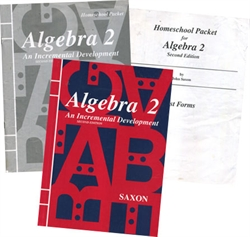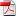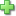# Saxon Algebra 2 - Home Study Kit (old)

Publisher: Saxon Publishers
2nd Edition, Item: 1685
Curriculum Bundle
Current Retail Price: \$65.00
Not in stock

See series description for full review.

Algebra 2 not only treats topics that are traditionally covered in second year algebra, but also a considerable amount of geometry. Specific topics include: graphical solution of simultaneous equations; scientific notation; radicals; roots of quadratic equations; properties of real numbers; inequalities and systems of inequalities; logarithms and antilogarithms; exponential equations; basic trigonometric functions; algebra of polynomials; vectors; polar and rectangular coordinate systems; and a wide spectrum of algebraic word problems. Time is also spent developing geometric concepts and writing proof outlines (the proof coverage is weak). Applications to other subjects such as physics and chemistry, plus "real world" problems, are also covered. (129 lessons)

The student edition contains the answers to the odd-numbered questions; the Home Study Packet, which is the complete answer key and tests, is also included. You will need a scientific calculator (Sharp recommended).

## Samples:Table of ContentsLesson 2Lesson 35Lesson 106Test 1Answer KeyClick here to write a review